# 【算法】KMP算法

## KMP算法

### 2. Brute-Force（暴力解法）

#### 2.2 代码实现：

public int BF(String s, String p){
int m = s.length();
int n = p.length();
for(int i = 0; i <= s-n; i++){  //控制主串比较的位置；
boolean flag = true;
for(int j = 0; j < n; j++){   //控制模式串比较的位置；每次都从头比较；
if(s.charAt(i+j) != p.charAt(j)){
break;
flag = false;
}
}
if(flag){
return i;
}
}
return -1;
}


### 3. KMP算法

#### 3.1 KMP匹配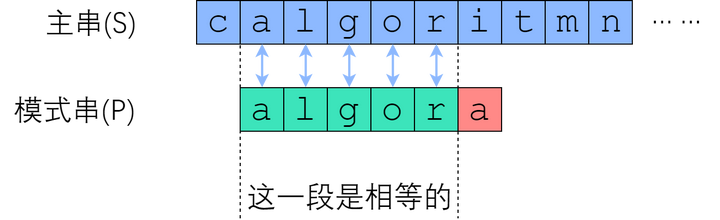##### 跳过不可能的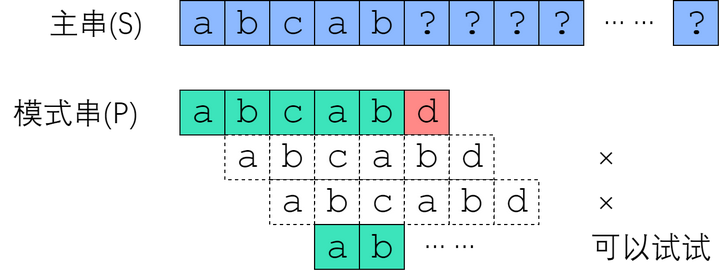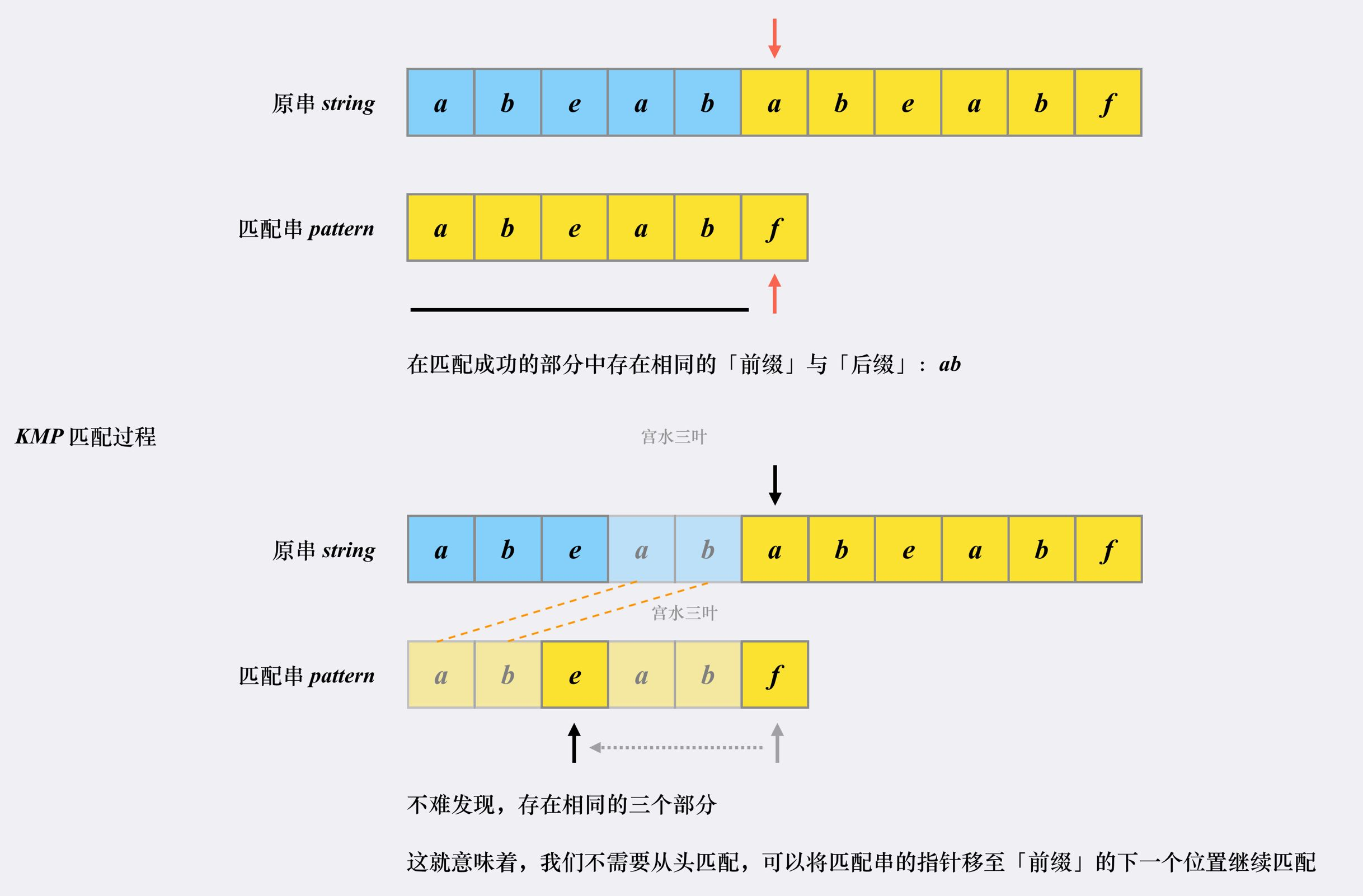KMP 为什么相比于朴素解法更快：

• 因为 KMP 利用已匹配部分中相同的「前缀」和「后缀」来加速下一次的匹配。
• 因为 KMP 的主串指针不会进行回溯（没有朴素匹配中回到下一个「发起点」的过程）。主串指针始终是在右移；其实是意味着：随着匹配过程的进行，原串指针的不断右移，我们本质上是在不断地在否决一些「不可能」的方案。

#### 3.2 next数组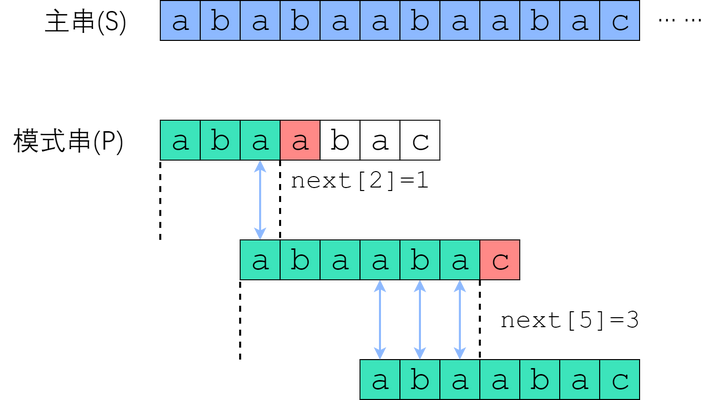• P[x] = P[now]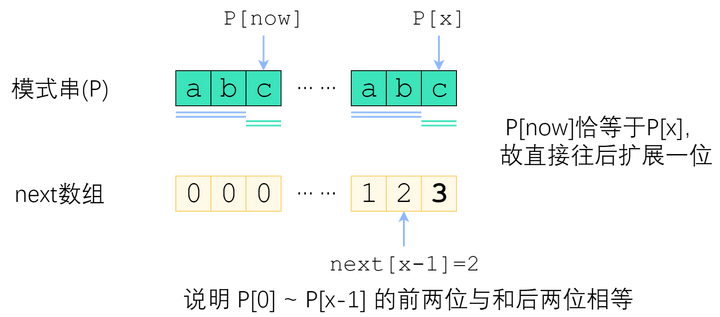• P[x] != P[now]
如图。长度为 now 的子串 A 和子串 B 是 P~P[x-1] 中最长的公共前后缀。可惜 A 右边的字符和 B 右边的那个字符不相等，next[x]不能改成 now+1 了。因此，我们应该缩短这个now，把它改成小一点的值，再来试试 P[x] 是否等于 P[now].
now该缩小到多少呢？显然，我们不想让now缩小太多。因此我们决定，在保持“P~P[x-1]的now-前缀仍然等于now-后缀”的前提下，让这个新的now尽可能大一点。 P~P[x-1] 的公共前后缀，前缀一定落在串A里面、后缀一定落在串B里面。换句话讲：接下来now应该改成：使得 A的k-前缀等于B的k-后缀 的最大的k.
可以利用的一个关键信息：串A和串B是相同的！所以B的后缀就等于A的后缀；因此，使得A的k-前缀等于B的k-后缀的最大的k，其实就是串A的最长公共前后缀的长度 —— next[now-1]！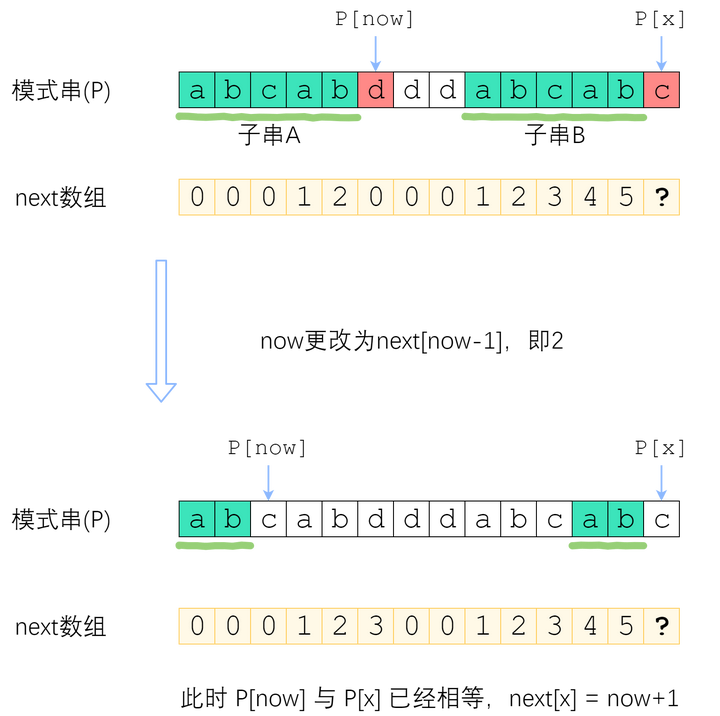#### 3.3 代码实现

public int KMP(String s, String p){
int tar = 0;
int pos = 0;
char[] arrs = s.toCharArray();
char[] arrp = p.toCharArray();
int m = s.length();
int n = p.length();
int[] next = buildNext(arrp, n);
while(tar < m){
if(arrs[tar] == arrp[pos]){
tar++;    //匹配则比较下一个元素；
pos++;
}else if(pos != 0){
pos = next[pos-1];   //不匹配且没回到头，回溯；
}else{
tar++;   //回到头证明此tar匹配不上了，右移；
}
if(pos == n){   //走完模式串后即匹配成功了；
return tar-pos;
}
}
return -1;
}

//构建next数组；
private int[] buildNext(char[] p, int n){
int[] next = new int[n];
int now = 0;
int i = 1;
while(i < n){
if(p[now] == p[i]){
now++;    //一致时填充next并移动指针；
next[i] = now;
i++;
}else if(now != 0){   //缩小now；
now = next[now-1];
}else{
next[i] == 0;   //now回到头了，还不一致，直接给next对应位置填0，接着右移；
i++;
}
}
return next;
}


KMP匹配时tar指针和构建next数组时i指针都是始终右移，没有回溯；

### 参考

posted @ 2021-07-15 10:18  Curryxin  阅读(101)  评论(0编辑  收藏  举报
Live2D